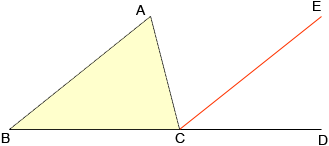Name: Rachel Who is asking: Student Level of the question: Middle Question: how do you prove, without knowing any of the measurements or degrees, that the three angles of a triangle equal 180? what are the steps for proving that? Hi Rachel, Suppose the triangle has vertices ABC, then extend one side (I extended BC) to a point D. Next draw a line segment through C parallel to the opposite side (here AB).Since AB and EC are parallel, and AC is a transversal, angle CAB is congruent to angle ACE. Since AB and EC are parallel, and BC is a transversal, angle ABC is congruent to angle ECD. Thus angle BCA + angle CAB + angle ABC = angle BCA + angle ACE + angle ECD But angle BCA + angle ACE + angle ECD = angle BCD = 180o Thus angle BCA + angle CAB + angle ABC = 180o Penny